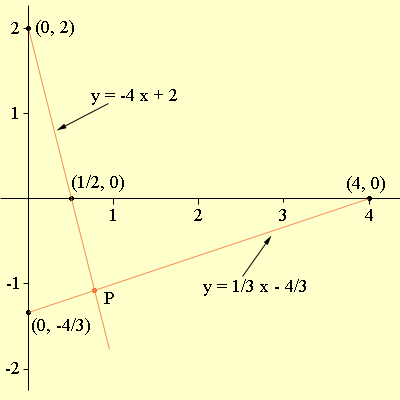SEARCH HOMEMath Central Quandaries & QueriesI was told to make a graph to help solve this question. I was given two equations. One was x-2y=3 and the other was 2x+y=-4. We have to change it to the equation of the line format. Then we graph the line and find the point where they meet. I've been trying to do that, but when i check the answer, It turns out wrong. It's meeting in the wrong spot. I need help please.Hi Tamara,

Let me try this with two slightly different equations,

x - 3y = 4
4x + y = 2

Changing these equations to the slope-intercept form I get

y = 1/3 x - 4/3
y = -4 x + 2

Now I plotted the line using the intercepts. In the first equation when x = 0, y = -4/3 and
when y = 0, x = 4. In the second equation when x = 0, y = 2 and when y = 0, x = 1/2.The point P is the intersection point and I can see for the diagram that the x-coordinate of P is a little less than 1 and the y-coordinate of P is a little less than -1. I then solved for P algebraically and found that P is (10/13, -14/13).

I hope this helps,
PennyMath Central is supported by the University of Regina and The Pacific Institute for the Mathematical Sciences.